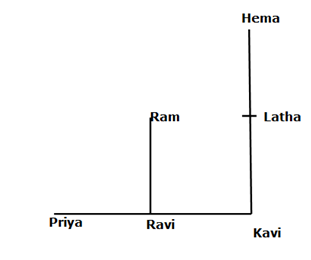# SBI Clerk Prelims Reasoning Ability Questions 2021 (Day-31)

Dear Aspirants, Our IBPS Guide team is providing new series of Reasoning Questions for SBI Clerk Prelims 2021 so the aspirants can practice it on a daily basis. These questions are framed by our skilled experts after understanding your needs thoroughly. Aspirants can practice these new series questions daily to familiarize with the exact exam pattern and make your preparation effective.

Start Quiz

Direction sense

Direction (1-3): Study the following information carefully and answer the below questions

In the school assembly, Ram is standing north of Ravi who stands exactly between Priya and Kavi. Latha stands north of Kavi and east of Ram. Hema stands north-east of Priya and north of Latha. The distance between the adjacent persons is the same.

1) What is the direction of Hema with respect to Ravi?

A.East

B.West

C.North-east

D.South

E.South-west

2) If Hema and Ravi interchange their positions and Ram and Kavi did the same, with respect to the new positions, who among the following stand exactly between Priya and Ram?

A.Kavi

B.Ravi

C.Hema

D.Ram

E.None of the above

3) Who among the following person is not stands to the south-west of Hema?

A.Ram

B.Ravi

C.Priya

D.Kavi

E.None of the above

Misc

4) If all the letters of the word “INTELLIGENT” are changed to the next successive letter then how many vowels are there in the word?

A.1

B.2

C.3

D.4

E.None

5) In the given number “671832945”, What is the sum of the cube value of the numbers which is fourth from the right end and third from the left end?

A.65

B.91

C.09

D.28

E.68

Inequality

Direction (6-9): In each of the following questions, the relationship between different elements is shown in the statements followed by two conclusions. Find the conclusion which is definitely true.

6) Statements:

A > D > R < T = F ≤ M < S > G

Conclusions:

I).  A > T

II). R < S

A.If only conclusion I is true.

B.If only conclusion II is true.

C.If either conclusion I or II is true.

D.If neither conclusion I nor II is true.

E.If both conclusions I and II are true.

7)
Statements:

N > Y > R = W > S ≤ V ≥ L = H

Conclusions:

I). N > S

II). H ≥ V

A.If only conclusion I is true.

B.If only conclusion II is true.

C.If either conclusion I or II is true.

D.If neither conclusion I nor II is true.

E.If both conclusions I and II are true.

8) Statements:

X > Y = Z ≥ Q ≥ R ≥ I = P > N

Conclusions:

I). Y > N

II). Z < P

A.If only conclusion I is true.

B.If only conclusion II is true.

C.If either conclusion I or II is true.

D.If neither conclusion I nor II is true.

E.If both conclusions I and II are true.

9) Statements:

S > R > E < H ≥ W ≥ T > A > M

Conclusions:

I). S < M

II). E > A

A.If only conclusion I is true.

B.If only conclusion II is true.

C.If either conclusion I or II is true.

D.If neither conclusion I nor II is true.

E.If both conclusions I and II are true.

Misc

10) If in the number 246357285, the digits at the odd position are increased by 1 and the digits at the even position are decreased by 2 from the left end, then in the number thus obtained how many digits are even numbers?

A.Two

B.Three

C.More than three

D.One

E.None

Directions (1-3):I N  T E L L  I G E N T

O U F M M J H F O U

671832945

13+23=1+8=9